# 3 DERIVATIVES DERIVATIVES In this chapter we begin

• Slides: 62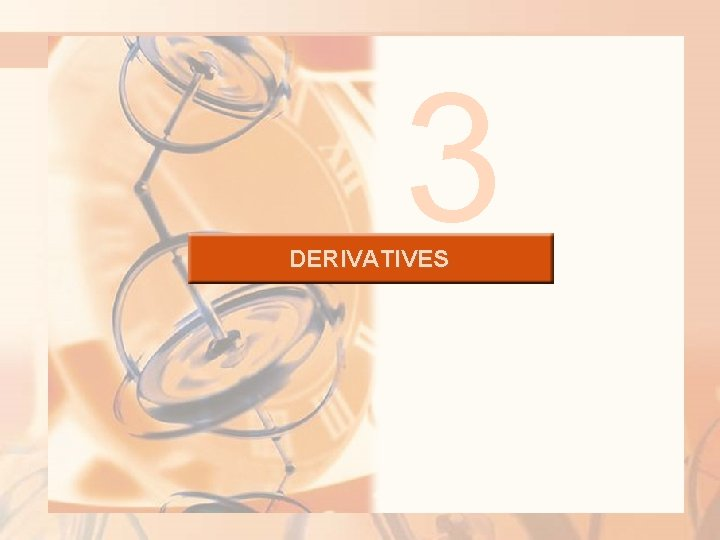3 DERIVATIVES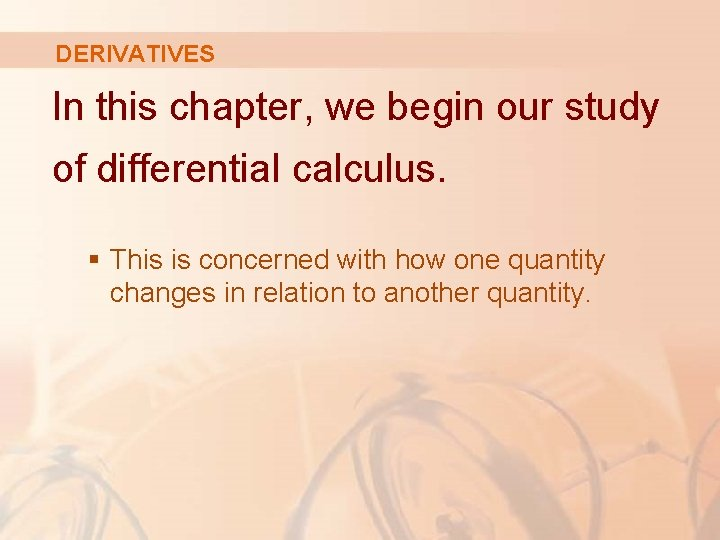DERIVATIVES In this chapter, we begin our study of differential calculus. § This is concerned with how one quantity changes in relation to another quantity.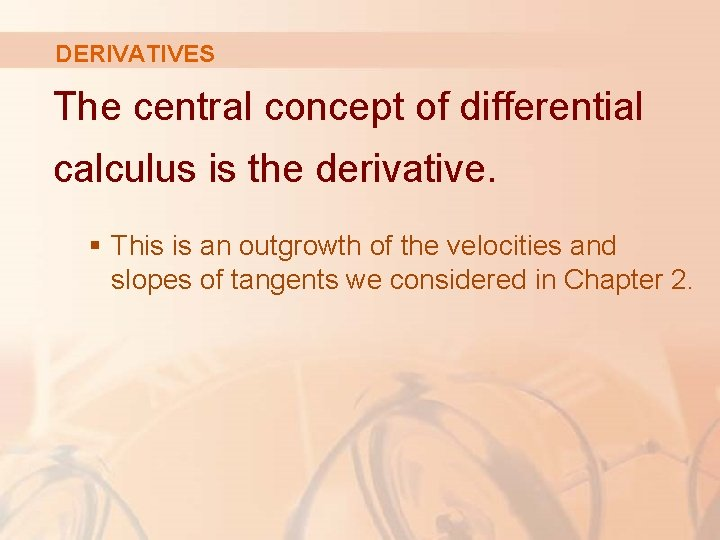DERIVATIVES The central concept of differential calculus is the derivative. § This is an outgrowth of the velocities and slopes of tangents we considered in Chapter 2.DERIVATIVES After learning how to calculate derivatives, we use them to solve problems involving: § Rates of change § Approximation of functionsDERIVATIVES 3. 1 Derivatives and Rates of Change In this section, we will learn: How the derivative can be interpreted as a rate of change in any of the sciences or engineering.DERIVATIVES AND RATES OF CHANGE The problem of finding the tangent line to a curve and the problem of finding the velocity of an object both involve finding the same type of limit. § This special type of limit is called a derivative. § We will see that it can be interpreted as a rate of change in any of the sciences or engineering.TANGENTS If a curve C has equation y = f(x) and we want to find the tangent line to C at the point P(a, f(a)), then we consider a nearby point Q(x, f(x)), where , and compute the slope of the secant line PQ: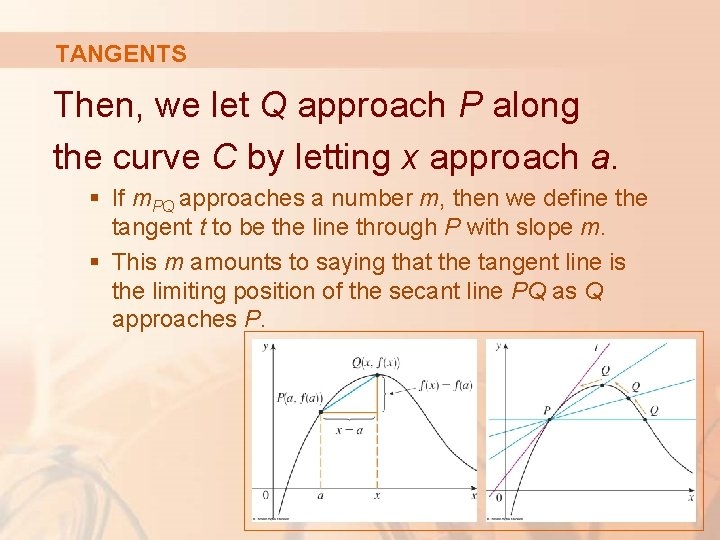TANGENTS Then, we let Q approach P along the curve C by letting x approach a. § If m. PQ approaches a number m, then we define the tangent t to be the line through P with slope m. § This m amounts to saying that the tangent line is the limiting position of the secant line PQ as Q approaches P.TANGENTS 1. Definition The tangent line to the curve y = f(x) at the point P(a, f(a)) is the line through P with slope provided that this limit exists.TANGENTS In our first example, we confirm the guess we made in Example 1 in Section 2. 1.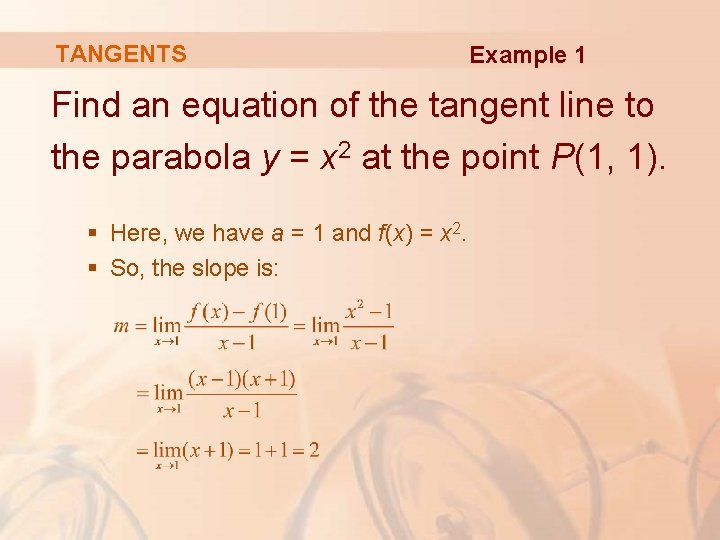TANGENTS Example 1 Find an equation of the tangent line to the parabola y = x 2 at the point P(1, 1). § Here, we have a = 1 and f(x) = x 2. § So, the slope is:TANGENTS Example 1 Using the point-slope form of the equation of a line, we find that an equation of the tangent line at (1, 1) is: y - 1 = 2(x - 1) or y = 2 x - 1TANGENTS We sometimes refer to the slope of the tangent line to a curve at a point as the slope of the curve at the point. § The idea is that, if we zoom in far enough toward the point, the curve looks almost like a straight line.TANGENTS The figures illustrate this procedure for the curve y = x 2 in Example 1. § The more we zoom in, the more the parabola looks like a line. § In other words, the curve becomes almost indistinguishable from its tangent line.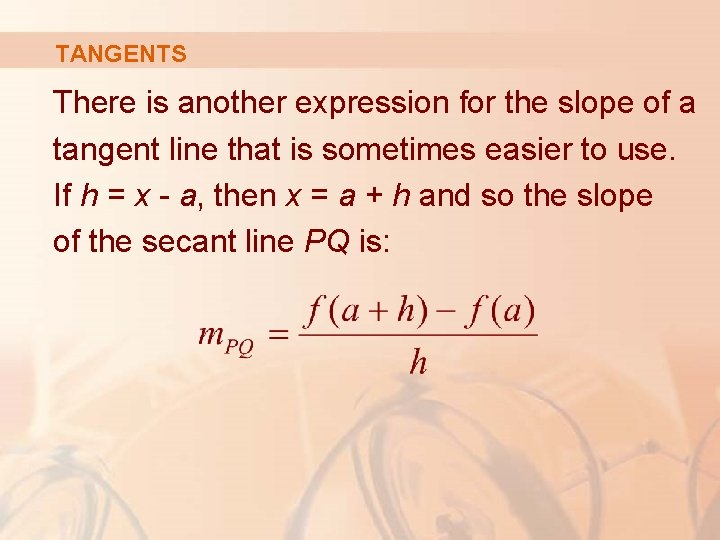TANGENTS There is another expression for the slope of a tangent line that is sometimes easier to use. If h = x - a, then x = a + h and so the slope of the secant line PQ is:TANGENTS In the figure, the case h > 0 is illustrated and Q is to the right of P. § If it happened that h < 0, Q would be to the left of P.TANGENTS 2. Definition Notice that, as x approaches a, h approaches 0 (because h = x - a). § So, the expression for the slope of the tangent line in Definition 1 becomes: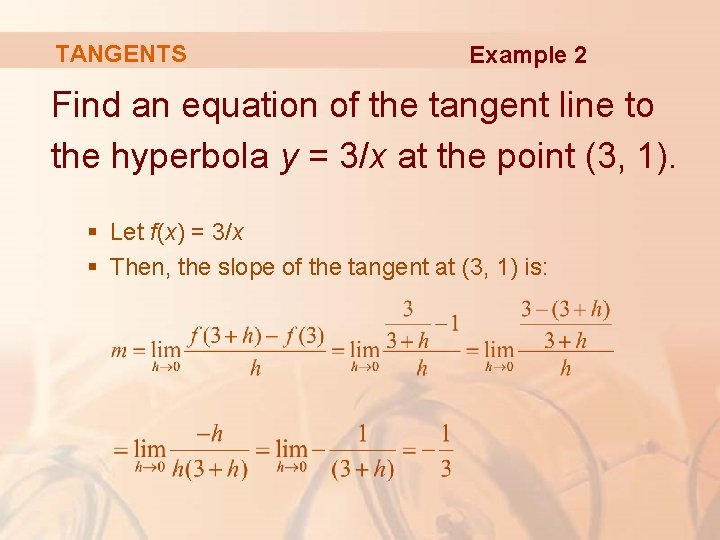TANGENTS Example 2 Find an equation of the tangent line to the hyperbola y = 3/x at the point (3, 1). § Let f(x) = 3/x § Then, the slope of the tangent at (3, 1) is: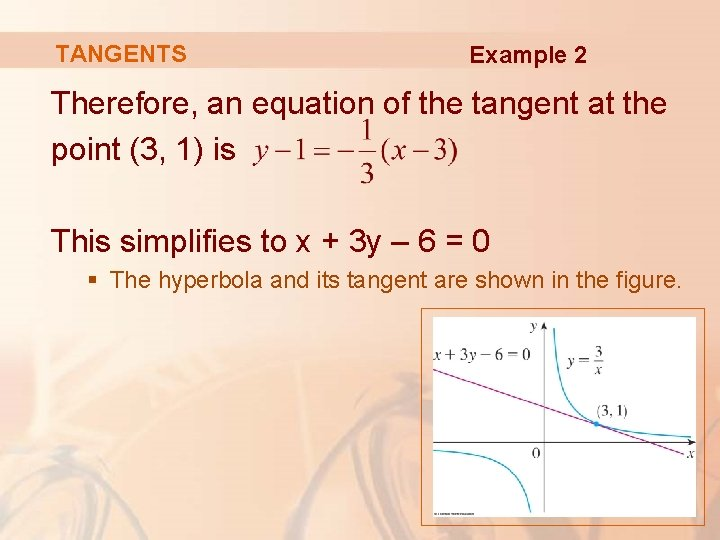TANGENTS Example 2 Therefore, an equation of the tangent at the point (3, 1) is This simplifies to x + 3 y – 6 = 0 § The hyperbola and its tangent are shown in the figure.VELOCITIES In general, suppose an object moves along a straight line according to an equation of motion s = f(t) § S is the displacement (directed distance) of the object from the origin at time t. § The function f that describes the motion is called the position function of the object.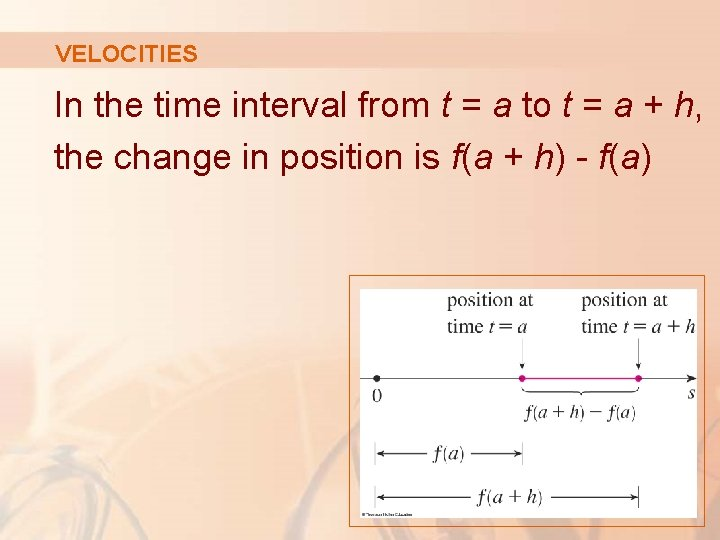VELOCITIES In the time interval from t = a to t = a + h, the change in position is f(a + h) - f(a)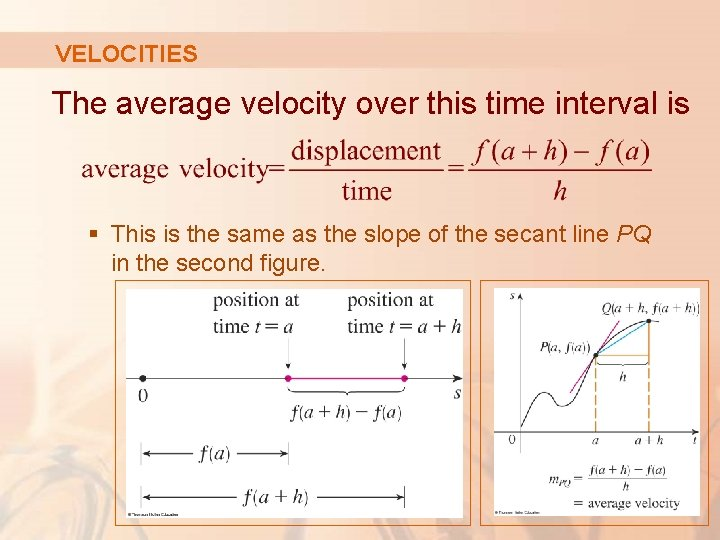VELOCITIES The average velocity over this time interval is § This is the same as the slope of the secant line PQ in the second figure.VELOCITIES Now, suppose we compute the average velocities over shorter and shorter time intervals [a, a + h]. § In other words, we let h approach 0.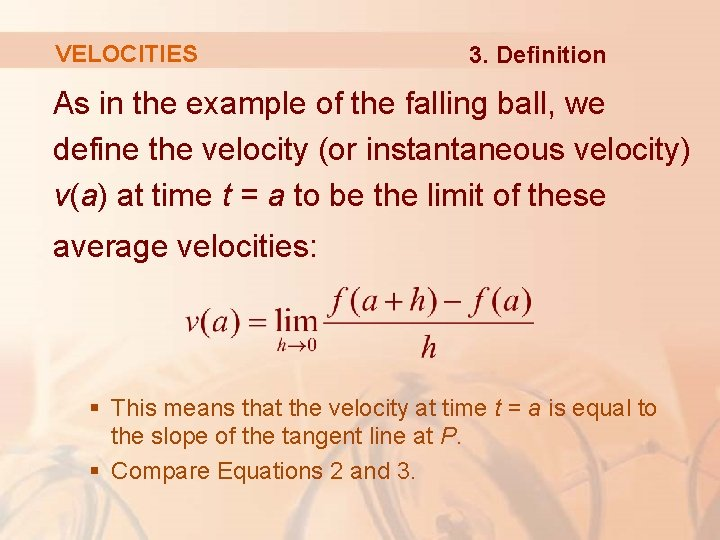VELOCITIES 3. Definition As in the example of the falling ball, we define the velocity (or instantaneous velocity) v(a) at time t = a to be the limit of these average velocities: § This means that the velocity at time t = a is equal to the slope of the tangent line at P. § Compare Equations 2 and 3.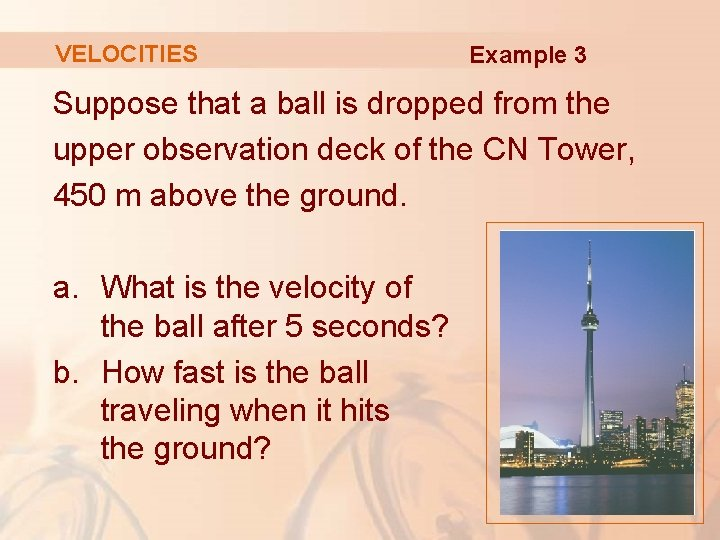VELOCITIES Example 3 Suppose that a ball is dropped from the upper observation deck of the CN Tower, 450 m above the ground. a. What is the velocity of the ball after 5 seconds? b. How fast is the ball traveling when it hits the ground?VELOCITIES Example 3 We will need to find the velocity both when t = 5 and when the ball hits the ground. § So, it’s efficient to start by finding the velocity at a general time t = a.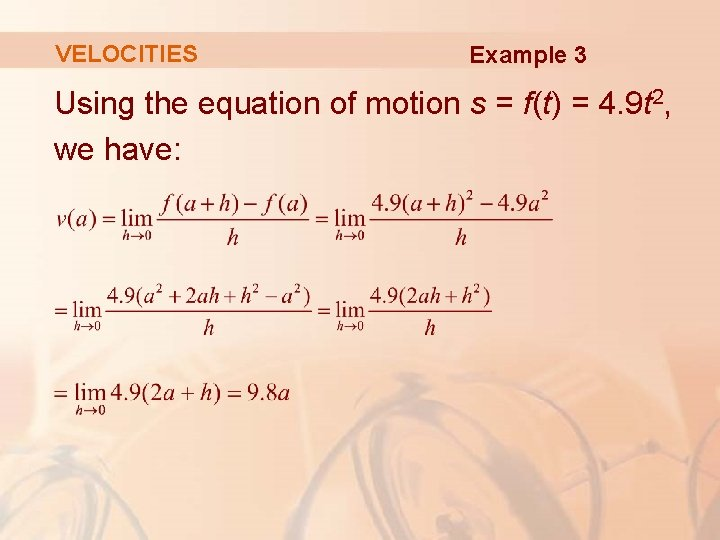VELOCITIES Example 3 Using the equation of motion s = f(t) = 4. 9 t 2, we have: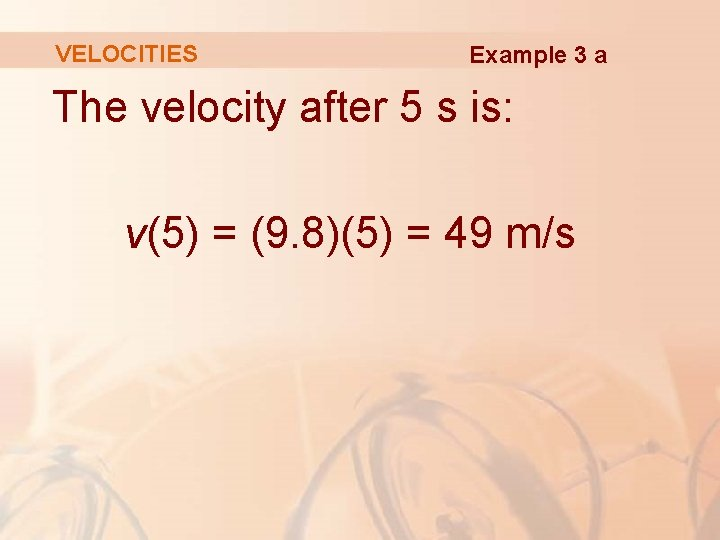VELOCITIES Example 3 a The velocity after 5 s is: v(5) = (9. 8)(5) = 49 m/s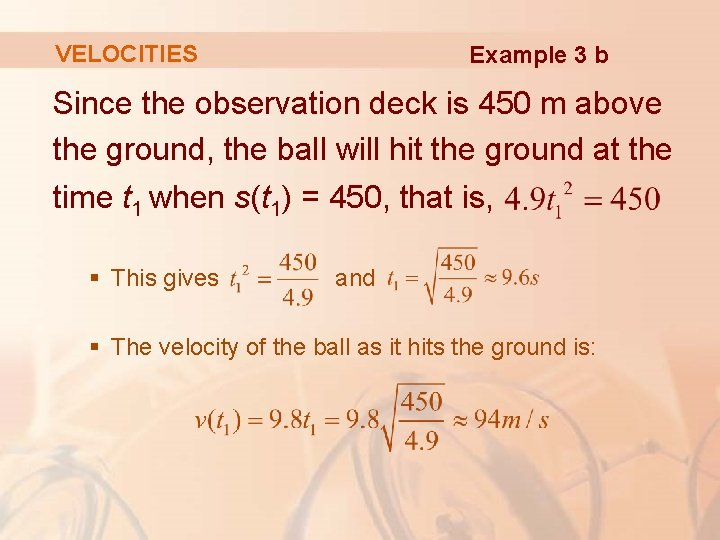VELOCITIES Example 3 b Since the observation deck is 450 m above the ground, the ball will hit the ground at the time t 1 when s(t 1) = 450, that is, § This gives and § The velocity of the ball as it hits the ground is: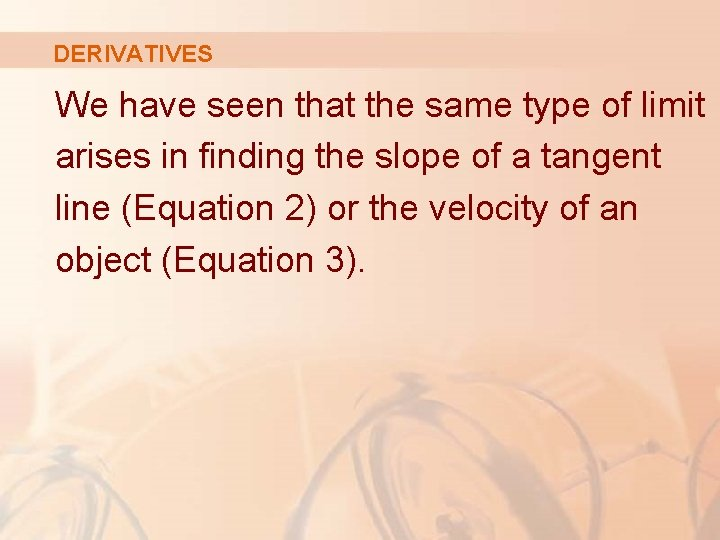DERIVATIVES We have seen that the same type of limit arises in finding the slope of a tangent line (Equation 2) or the velocity of an object (Equation 3).DERIVATIVES In fact, limits of the form arise whenever we calculate a rate of change in any of the sciences or engineering— such as a rate of reaction in chemistry or a marginal cost in economics. § Since this type of limit occurs so widely, it is given a special name and notation.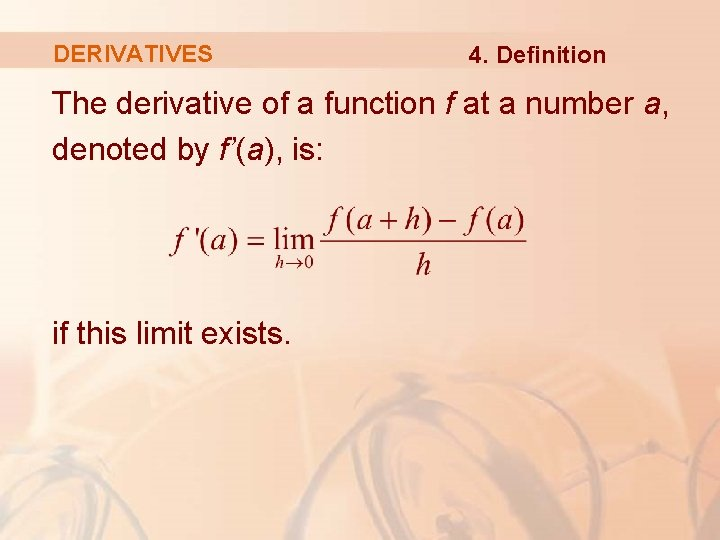DERIVATIVES 4. Definition The derivative of a function f at a number a, denoted by f’(a), is: if this limit exists.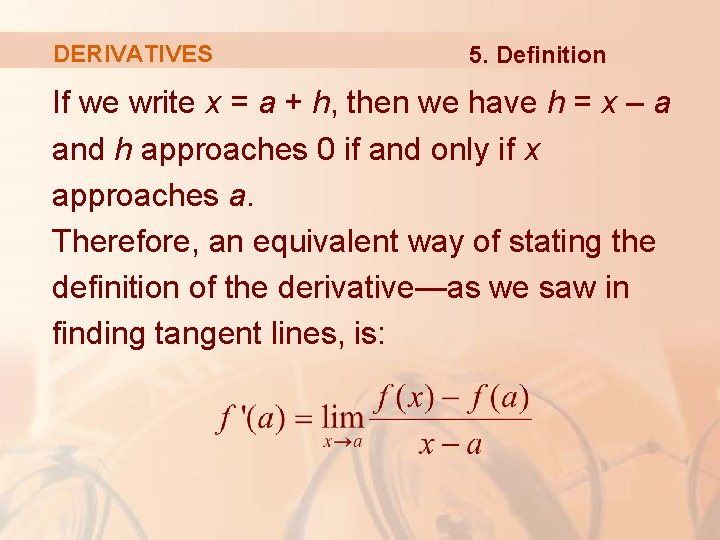DERIVATIVES 5. Definition If we write x = a + h, then we have h = x – a and h approaches 0 if and only if x approaches a. Therefore, an equivalent way of stating the definition of the derivative—as we saw in finding tangent lines, is:DERIVATIVES Example 4 Find the derivative of the function f(x) = x 2 - 8 x + 9 at the number a. § From Definition 4, we have:DERIVATIVES If we use the point-slope form of the equation of a line, we can write an equation of the tangent line to the curve y = f(x) at the point (a, f(a)): y - f(a) = f’(a)(x - a)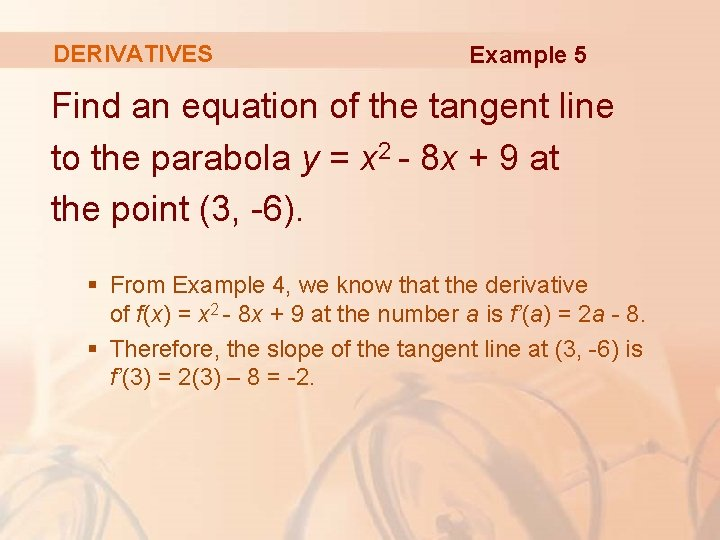DERIVATIVES Example 5 Find an equation of the tangent line to the parabola y = x 2 - 8 x + 9 at the point (3, -6). § From Example 4, we know that the derivative of f(x) = x 2 - 8 x + 9 at the number a is f’(a) = 2 a - 8. § Therefore, the slope of the tangent line at (3, -6) is f’(3) = 2(3) – 8 = -2.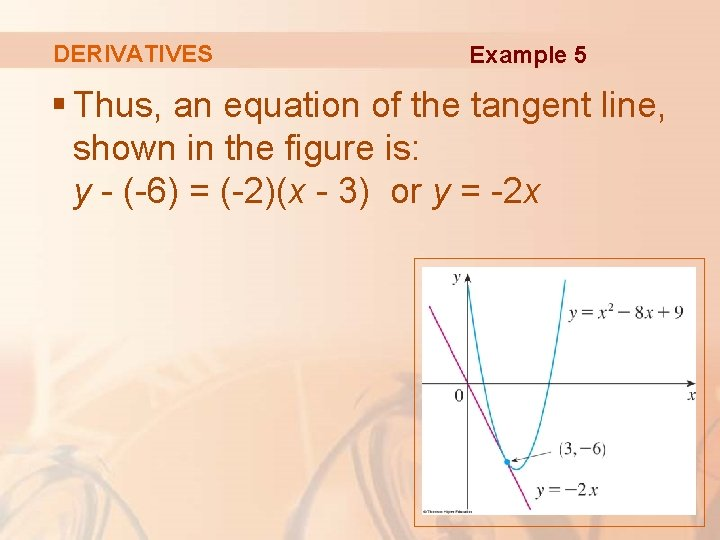DERIVATIVES Example 5 § Thus, an equation of the tangent line, shown in the figure is: y - (-6) = (-2)(x - 3) or y = -2 x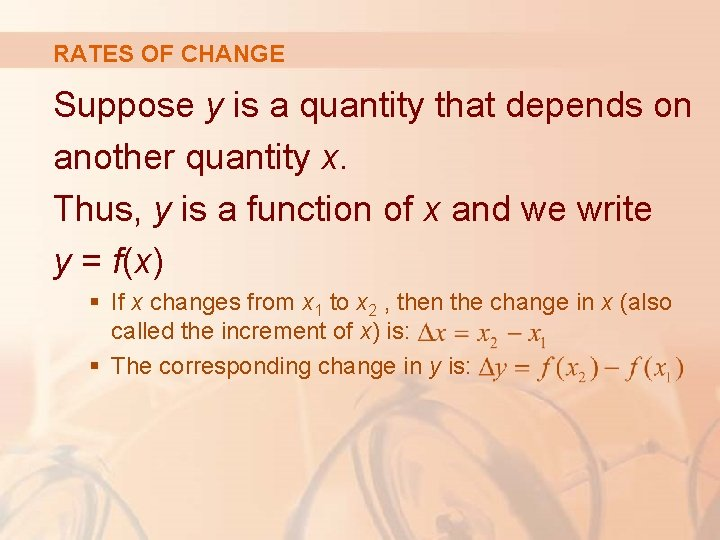RATES OF CHANGE Suppose y is a quantity that depends on another quantity x. Thus, y is a function of x and we write y = f(x) § If x changes from x 1 to x 2 , then the change in x (also called the increment of x) is: § The corresponding change in y is: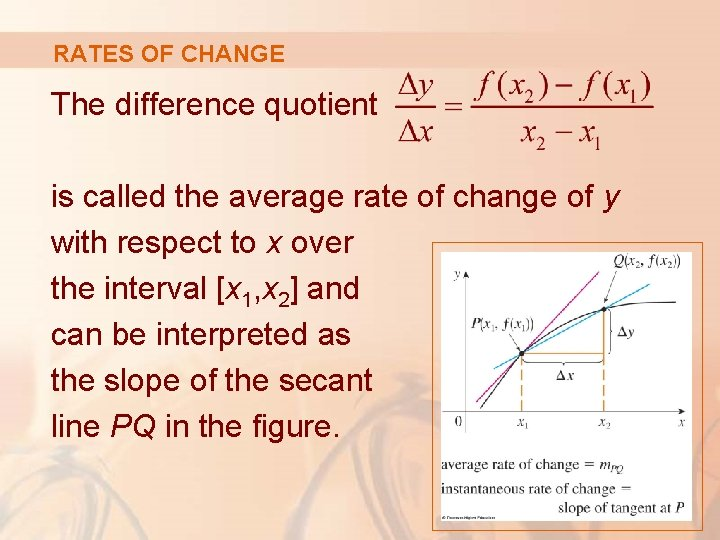RATES OF CHANGE The difference quotient is called the average rate of change of y with respect to x over the interval [x 1, x 2] and can be interpreted as the slope of the secant line PQ in the figure.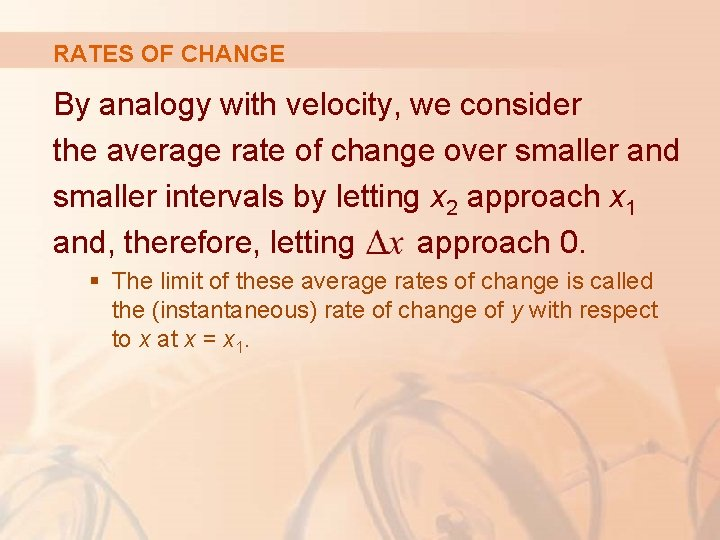RATES OF CHANGE By analogy with velocity, we consider the average rate of change over smaller and smaller intervals by letting x 2 approach x 1 and, therefore, letting approach 0. § The limit of these average rates of change is called the (instantaneous) rate of change of y with respect to x at x = x 1.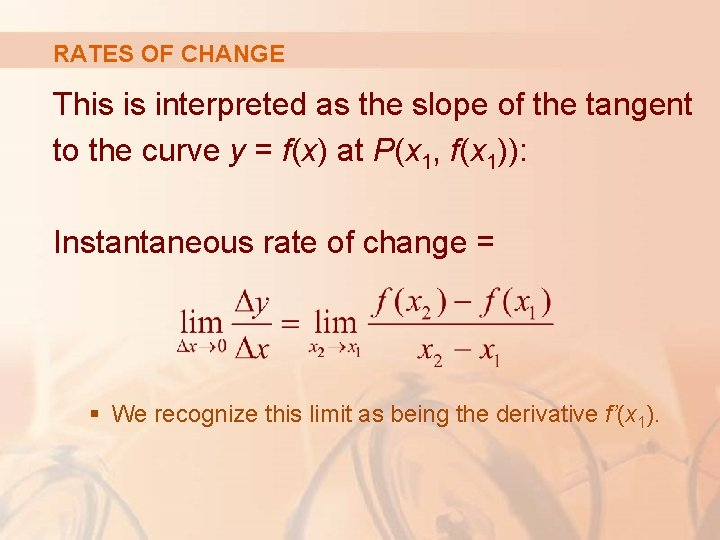RATES OF CHANGE This is interpreted as the slope of the tangent to the curve y = f(x) at P(x 1, f(x 1)): Instantaneous rate of change = § We recognize this limit as being the derivative f’(x 1).RATES OF CHANGE We know that one interpretation of the derivative f’(a) is as the slope of the tangent line to the curve y = f(x) when x = a. We now have a second interpretation. § The derivative f’(a) is the instantaneous rate of change of y = f(x) with respect to x when x = a.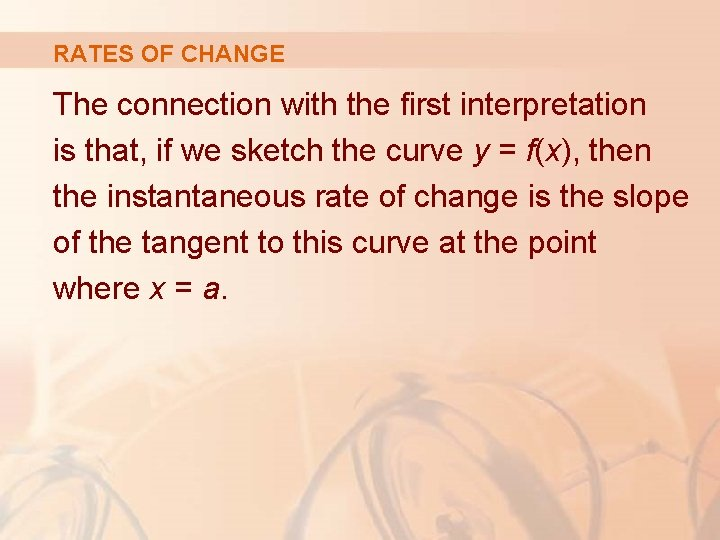RATES OF CHANGE The connection with the first interpretation is that, if we sketch the curve y = f(x), then the instantaneous rate of change is the slope of the tangent to this curve at the point where x = a.RATES OF CHANGE This means that: § When the derivative is large (and therefore the curve is steep, as at the point P in the figure), the y-values change rapidly. § When the derivative is small, the curve is relatively flat and the y-values change slowly.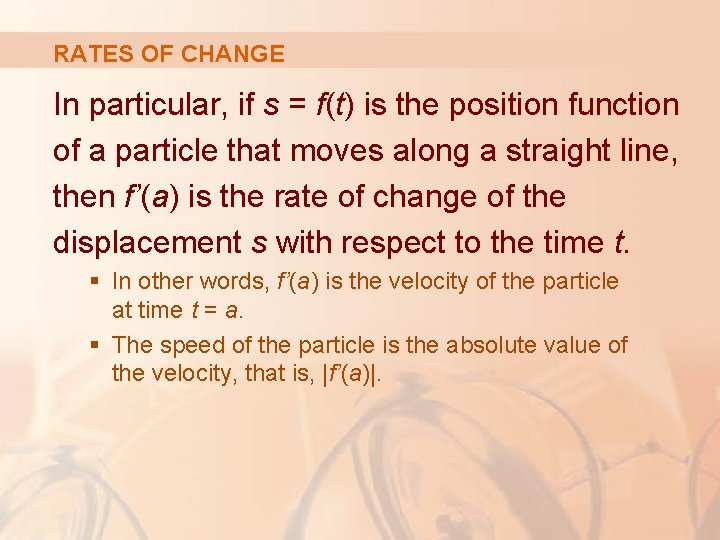RATES OF CHANGE In particular, if s = f(t) is the position function of a particle that moves along a straight line, then f’(a) is the rate of change of the displacement s with respect to the time t. § In other words, f’(a) is the velocity of the particle at time t = a. § The speed of the particle is the absolute value of the velocity, that is, |f’(a)|.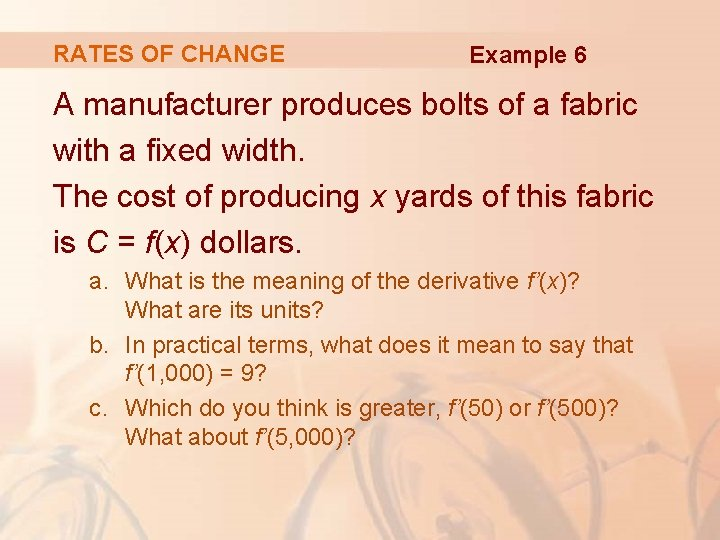RATES OF CHANGE Example 6 A manufacturer produces bolts of a fabric with a fixed width. The cost of producing x yards of this fabric is C = f(x) dollars. a. What is the meaning of the derivative f’(x)? What are its units? b. In practical terms, what does it mean to say that f’(1, 000) = 9? c. Which do you think is greater, f’(50) or f’(500)? What about f’(5, 000)?RATES OF CHANGE Example 6 a The derivative f’(x) is the instantaneous rate of change of C with respect to x. That is, f’(x) means the rate of change of the production cost with respect to the number of yards produced. § Economists call this rate of change the marginal cost.RATES OF CHANGE Example 6 a As the units for f’(x) are the same as the units for the difference quotient § Since is measured in dollars and in yards, it follows that the units for f’(x) are dollars per yard. .RATES OF CHANGE Example 6 b The statement that f’(1, 000) = 9 means that, after 1, 000 yards of fabric have been manufactured, the rate at which the production cost is increasing is \$9/yard. § When x =1, 000, C is increasing 9 times as fast as x.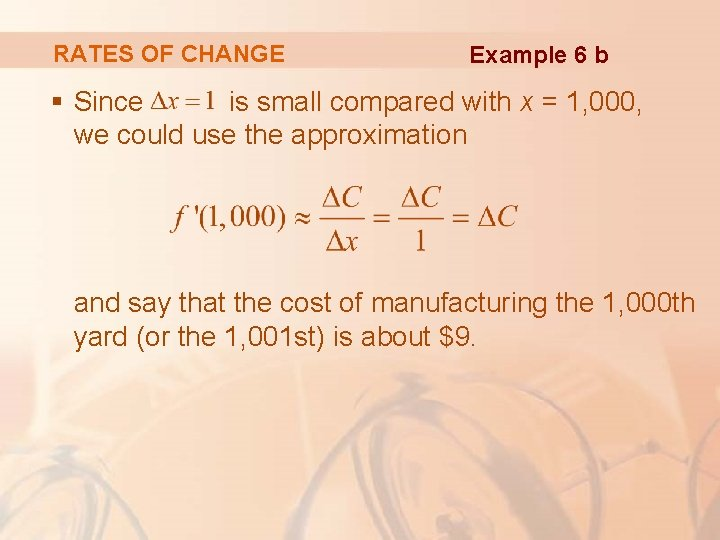RATES OF CHANGE Example 6 b § Since is small compared with x = 1, 000, we could use the approximation and say that the cost of manufacturing the 1, 000 th yard (or the 1, 001 st) is about \$9.RATES OF CHANGE Example 6 c The rate at which the production cost is increasing (per yard) is probably lower when x = 500 than when x = 50 (the cost of making the 500 th yard is less than the cost of the 50 th yard) due to economies of scale. § The manufacturer makes more efficient use of the fixed costs of production. § So,RATES OF CHANGE Example 6 c However, as production expands, the resulting large-scale operation might become inefficient and there might be overtime costs. § Thus, it is possible that the rate of increase of costs will eventually start to rise. § So, it may happen thatRATES OF CHANGE In the following example, we estimate the rate of change of the national debt with respect to time. § Here, the function is defined not by a formula but by a table of values.RATES OF CHANGE Example 7 Let D(t) be the US national debt at time t. The table gives approximate values of this function by providing end-of-year estimates, in billions of dollars, from 1980 to 2000. Interpret and estimate the value of D’(1990).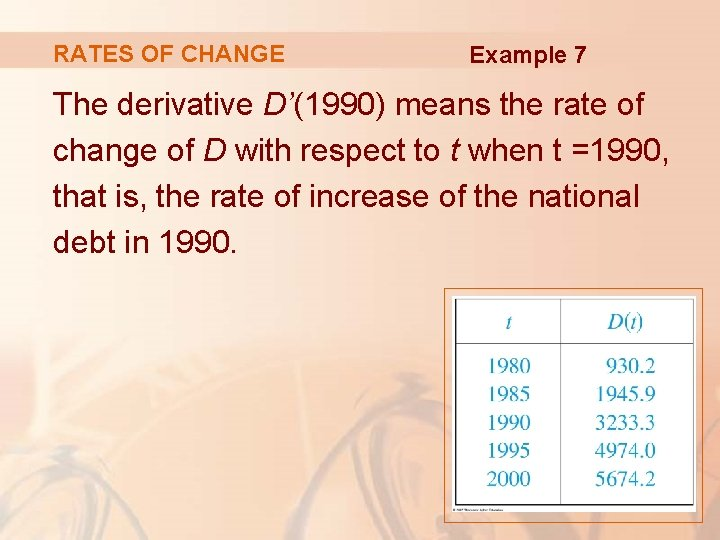RATES OF CHANGE Example 7 The derivative D’(1990) means the rate of change of D with respect to t when t =1990, that is, the rate of increase of the national debt in 1990.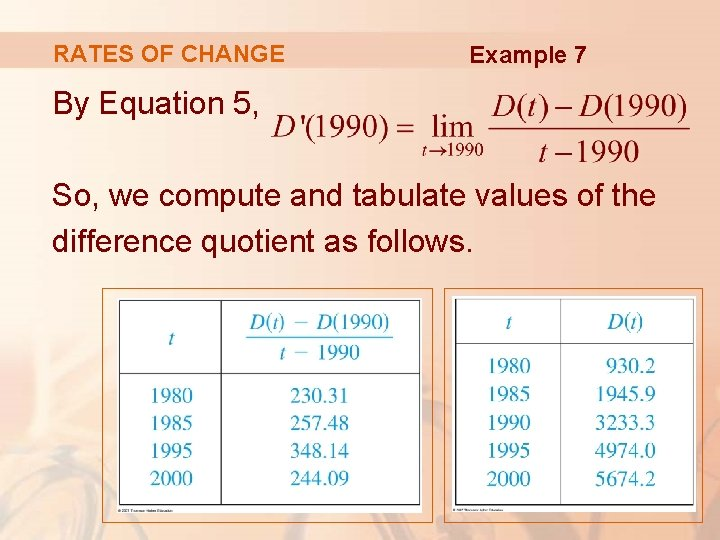RATES OF CHANGE Example 7 By Equation 5, So, we compute and tabulate values of the difference quotient as follows.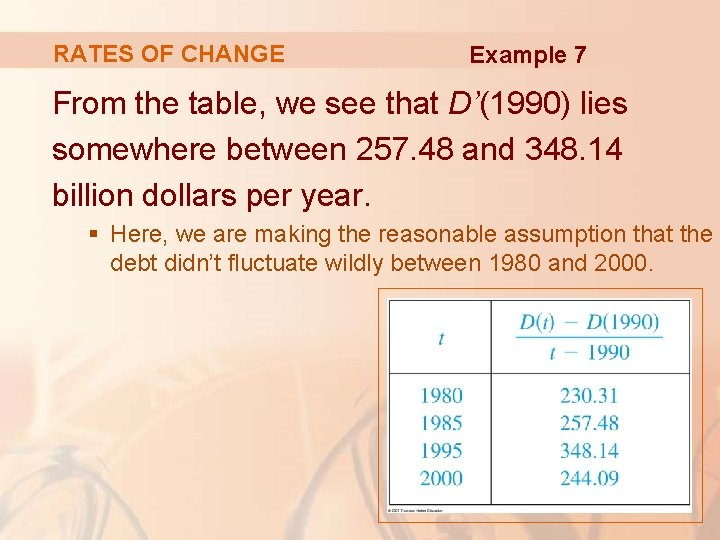RATES OF CHANGE Example 7 From the table, we see that D’(1990) lies somewhere between 257. 48 and 348. 14 billion dollars per year. § Here, we are making the reasonable assumption that the debt didn’t fluctuate wildly between 1980 and 2000.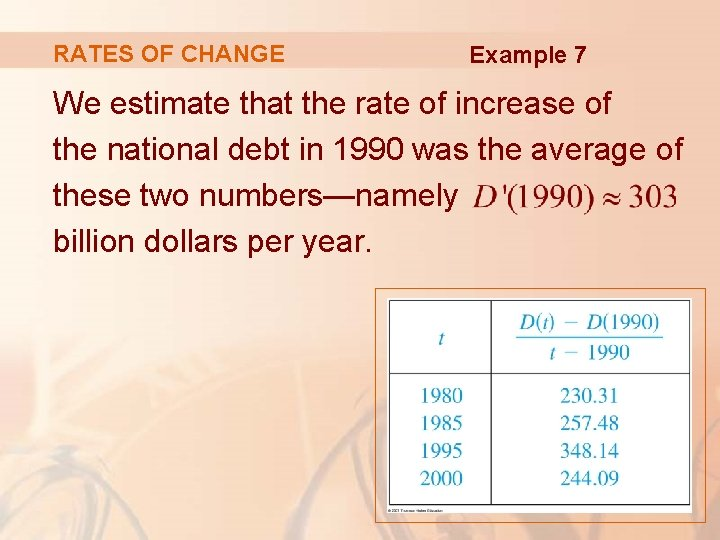RATES OF CHANGE Example 7 We estimate that the rate of increase of the national debt in 1990 was the average of these two numbers—namely billion dollars per year.RATES OF CHANGE Example 7 Another method would be to plot the debt function and estimate the slope of the tangent line when t = 1990.RATES OF CHANGE In Examples 3, 6, and 7, we saw three specific examples of rates of change: § The velocity of an object is the rate of change of displacement with respect to time. § The marginal cost is the rate of change of production cost with respect to the number of items produced. § The rate of change of the debt with respect to time is of interest in economics.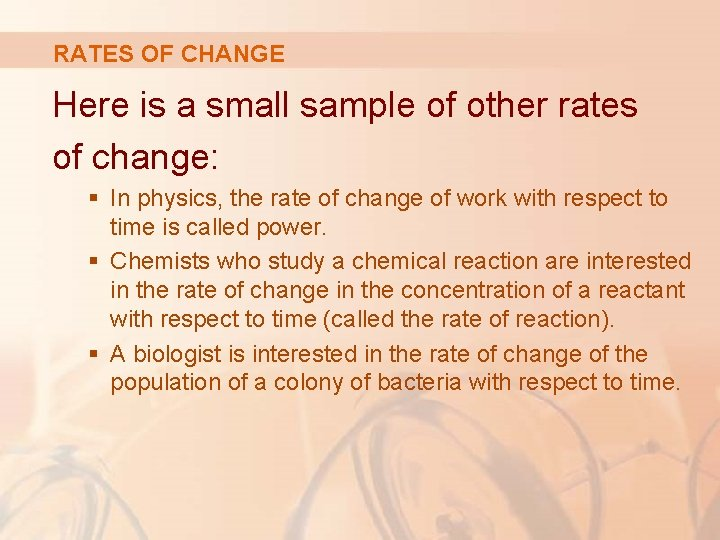RATES OF CHANGE Here is a small sample of other rates of change: § In physics, the rate of change of work with respect to time is called power. § Chemists who study a chemical reaction are interested in the rate of change in the concentration of a reactant with respect to time (called the rate of reaction). § A biologist is interested in the rate of change of the population of a colony of bacteria with respect to time.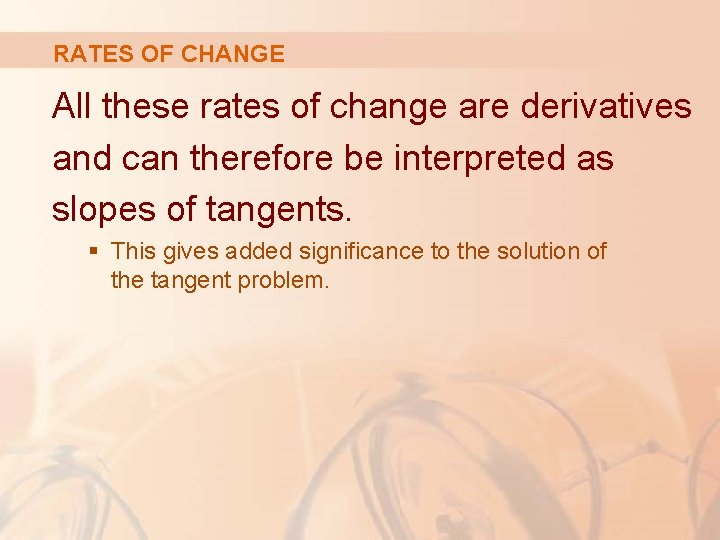RATES OF CHANGE All these rates of change are derivatives and can therefore be interpreted as slopes of tangents. § This gives added significance to the solution of the tangent problem.